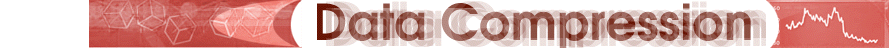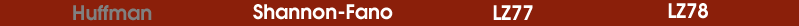Statistical Compressors Concept Algorithm Example Comparison (H vs. SF) The AlgorithmThe process used to create this tree is simple yet elegant: First count the amount of times each character appears. This is the frequency of each character. Create a collection of n small, one-node trees (where n is the number of distinct characters in the input stream). Each of these n trees represent a distinct input character and have a weight corresponding to their count tallied in the analysis step. From the collection, pick out the two trees with the smallest weights and remove them. Combine them into a new tree whose root has the weight equal to the sum of the weights of the two trees and with the two trees as its left and right subtrees. Add the new combined tree back into the collection. Continue this process - select the two trees (with anywhere from 1 to (n - 1) nodes) with lowest weight, join these by a new root node, set the root node's weight, and place the new tree back into the pool. Repeat this process until one tree encompassing all the input weights has been constructed. If at any point there is more than one way to choose the two trees of smallest weight, the algorithm chooses arbitrarily. The resultant large tree with a single root node is called a Huffman tree. This way, the nodes with the highest weight will be near the top of the tree, and have shorter codes. Next step: An example